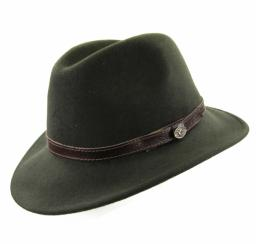# Probability 4020

There are numbers from 1 to 20 in the hat. What is the probability that we will pull out from the hat:

a / one-digit number
b / prime number
c / number greater than 11
d / a number divisible by six
Thank you

a =  0.45
b =  0.35
c =  0.45
d =  0.15

### Step-by-step explanation:Did you find an error or inaccuracy? Feel free to write us. Thank you!

Tips for related online calculators
Would you like to compute the count of combinations?